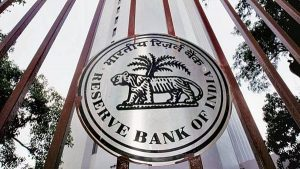# Floating Interest Rate : RBI

### Floating Interest Rate : RBIThe Reserve Bank of India (RBI) recently issued detailed guidelines to reset floating-interest rates on Equated Monthly Instalments (EMI)-based personal loans.

• Floating Interest Rate is an interest rate that changes periodically.
• The rate of interest moves up and down, or “floats,” reflecting economic or financial market conditions.
• A floating interest rate can also be referred to as an adjustable or variable interest rate because it can vary over the term of a debt obligation.
• The change in interest rate with a floating rate loan is typically based on a reference, or “benchmark”, rate that is outside of any control by the parties involved in the contract.
• The reference rate is usually a recognized benchmark interest rate, such as the prime rate, which is the lowest rate that commercial banks charge their most creditworthy customers for loans (typically, large corporations or high net worth individuals).
• A floating interest rate uses a reference rate as the base.
• In order to arrive at the floating rate, a spread (or margin) is added to the reference rate.
• Floating Interest Rate = Base Rate + Spread
• Floating interest rates can be modified quarterly, half-yearly or annually.
• Several factors tend to influence the calculation of floating interest rates. Some of the economic factors are,
• Repo rate
• Government’s monetary policies
• Inflation rate
• Fiscal deficit
• Global and foreign interest